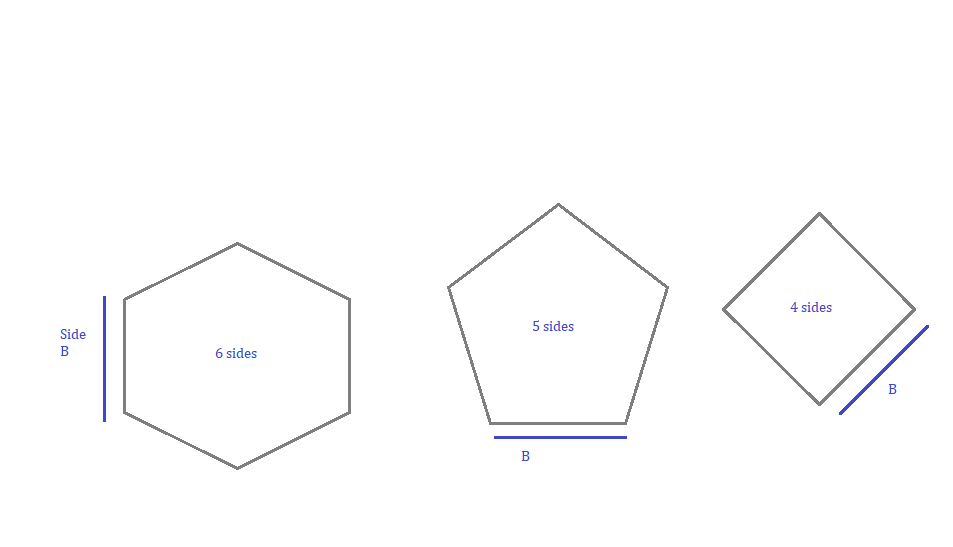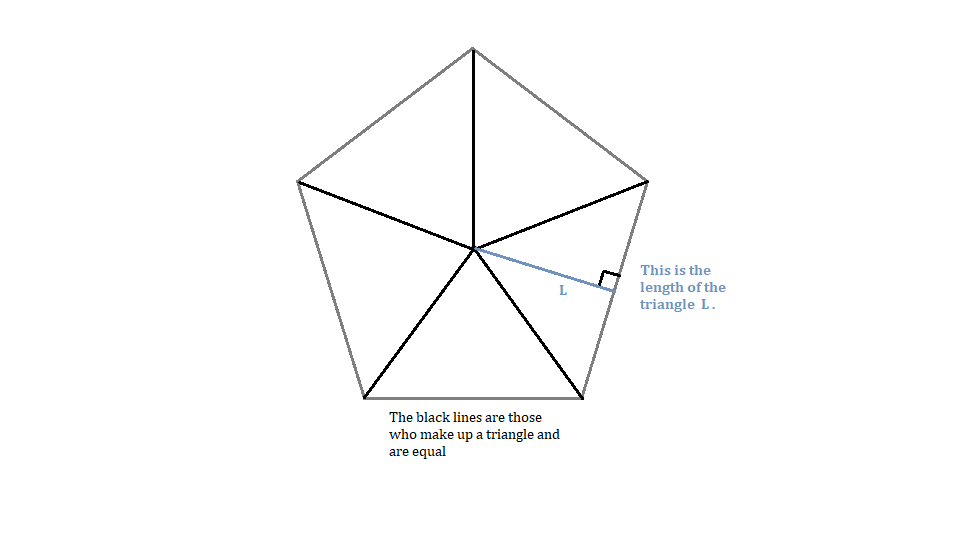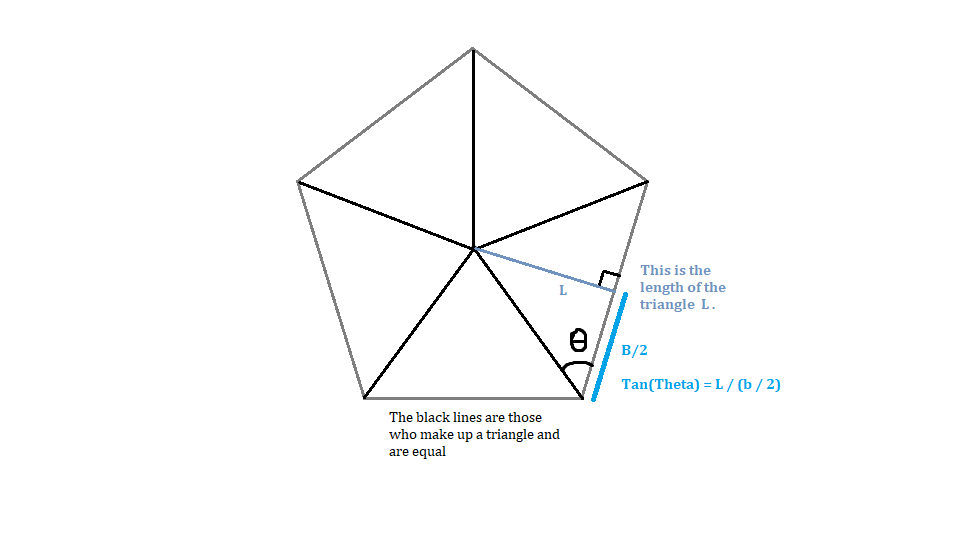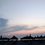# This is what keeps me up at 2 o'clock in the morning

I want to write the formula that gives the area of a regular polygon with base $b$ and no. of angles $n$. See the figure I made [made on Paint Mircosoft]!We can compose any polygon made of triangles, which always have 2 equal sides (Hexagon has 60-60-60 triangles, but it fits here), and the length of the triangle $l$ perpendicular to the base.We can start to say that the area of the polygon is:

$S_{polygon} = \frac{ b \times l }{ 2 } \times n$ $\text{ "n" is the no. of triangles in the polygon}$

We can express $l$ with trigonometry:• $\frac{ \tan \theta \times b }{ 2 } = l$

We replace this at the first formula:

• $S_{polygon} = \frac{ b \times \frac{ \tan \theta \times b }{ 2 } }{ 2 } \times n$
• $S_{polygon} = \frac{ b^2 \times \tan \theta \times n }{ 4 }$

How can we define $\theta$?

Remember that the sides of the regular polygon can be found using the formula $angle = \frac{(n - 2) \times 180 }{n}$, and for this case, it should be $\theta = \frac{(n - 2) \times 180 }{n \times 2}$, and, now we have the full formula:

$\boxed{ S_{polygon} = \frac{ b^2 \times \tan (\frac{(n - 2) \times 90 }{n}) \times n }{ 4 } }$

Please correct me for any mistake, typo or anything else against the guidelines. Ciao!Note by Nikolas Кraj
1 year, 5 months ago

This discussion board is a place to discuss our Daily Challenges and the math and science related to those challenges. Explanations are more than just a solution — they should explain the steps and thinking strategies that you used to obtain the solution. Comments should further the discussion of math and science.

When posting on Brilliant:

• Use the emojis to react to an explanation, whether you're congratulating a job well done , or just really confused .
• Ask specific questions about the challenge or the steps in somebody's explanation. Well-posed questions can add a lot to the discussion, but posting "I don't understand!" doesn't help anyone.
• Try to contribute something new to the discussion, whether it is an extension, generalization or other idea related to the challenge.

MarkdownAppears as
*italics* or _italics_ italics
**bold** or __bold__ bold
- bulleted- list
• bulleted
• list
1. numbered2. list
1. numbered
2. list
Note: you must add a full line of space before and after lists for them to show up correctly
paragraph 1paragraph 2

paragraph 1

paragraph 2

[example link](https://brilliant.org)example link
> This is a quote
This is a quote
    # I indented these lines
# 4 spaces, and now they show
# up as a code block.

print "hello world"
# I indented these lines
# 4 spaces, and now they show
# up as a code block.

print "hello world"
MathAppears as
Remember to wrap math in $$ ... $$ or $ ... $ to ensure proper formatting.
2 \times 3 $2 \times 3$
2^{34} $2^{34}$
a_{i-1} $a_{i-1}$
\frac{2}{3} $\frac{2}{3}$
\sqrt{2} $\sqrt{2}$
\sum_{i=1}^3 $\sum_{i=1}^3$
\sin \theta $\sin \theta$
\boxed{123} $\boxed{123}$

Sort by:

One thing I might add during my work, can the Brilliant team enable those features of editing with $LaTeX$ in creating notes/discussions, like those in creating problems or posting solutions to a problem?

- 1 year, 5 months ago

you can actually simplify the expression tan(((n-2)*90)/n) to tan(90-180/n) which reduces to (tan(180/n)^-1

- 1 year, 4 months ago

@Nikolas Kraj, you wrote spelling of "polygon" as "poligon"

- 1 year, 3 months ago

Thanks, I edited it. Please next time give a hint of where I made a typo (f.ex. you spelled side wrong at "... the siles in the polygon ... ".).

- 1 year, 3 months ago

Help at http://essaypapers.reviews/

- 1 year, 3 months ago

What is best for high quality party supplies as i am arranging a party and don't know from where I can buy.

- 1 year, 4 months ago